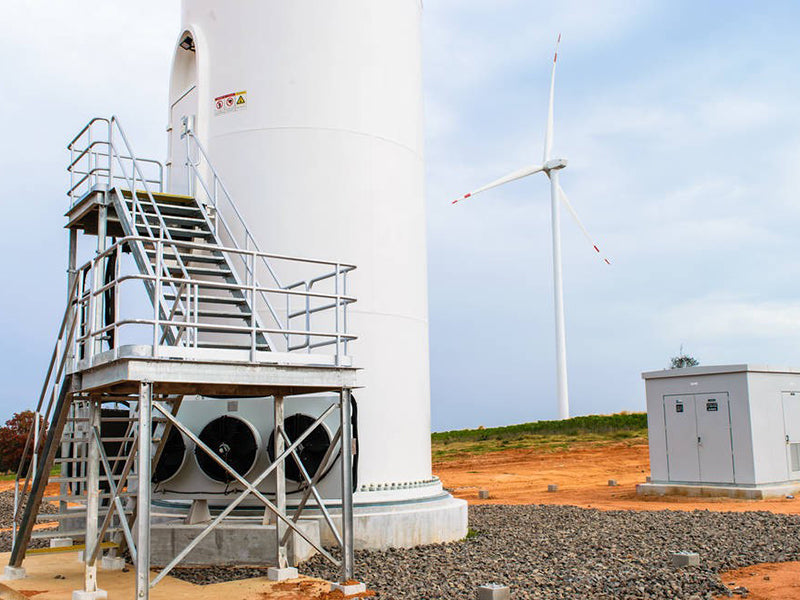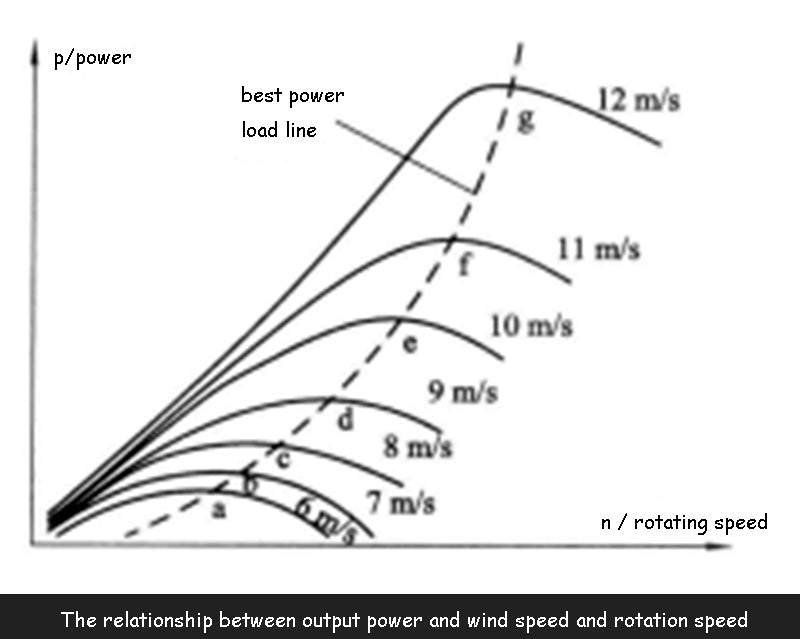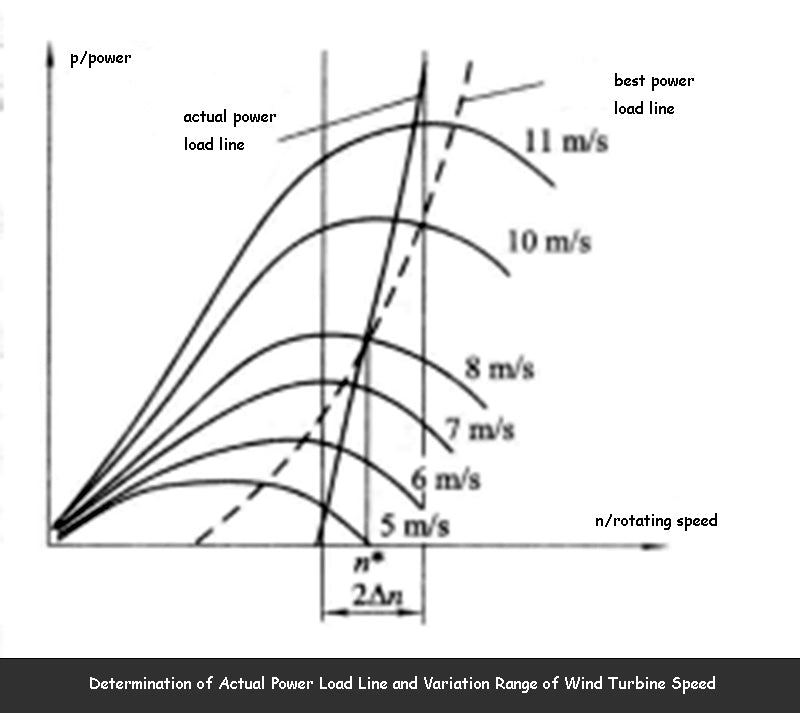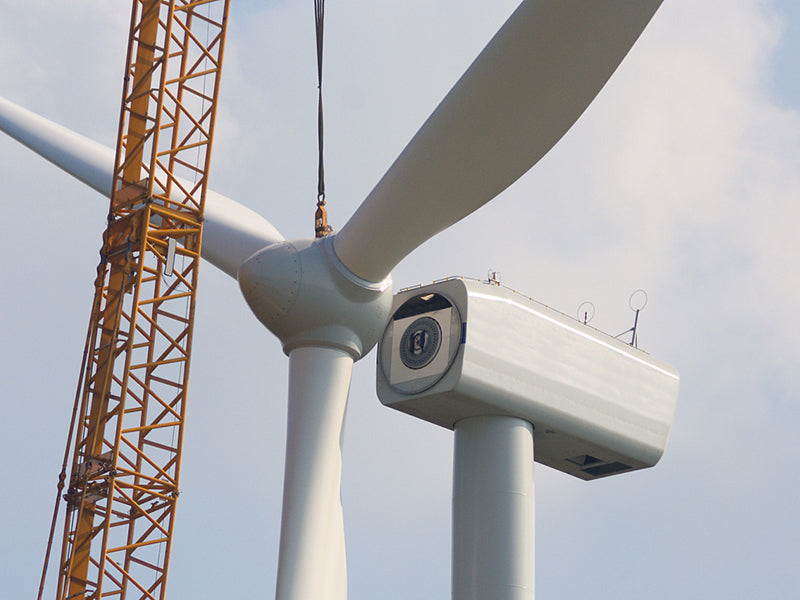## 1. Optimum power load line

At different wind speeds, the relationship between the output power of the wind turbine and the rotational speed of the wind turbine is shown in the figure below. It can be seen from the curve in the figure that under different wind speeds, there is a maximum output power in the characteristic curve of the relationship between the output power and the rotational speed of the wind turbine.power value, such as points a, b.c...g, and connect these points to the power load line to get the optimal power output line of the wind turbine, that is, the optimal power load line, as shown by the dotted line in the above figure. The power coefficient Cis the number of the high-speed coefficient (or tip speed ratio) λ of the wind turbine, that is, Cp=f(λ). When A=ω R/ν changes, the Cp value changes. At a certain λ value, the Cp value reaches the maximum value, and the λ value corresponding to this maximum Cp value(CP max)is called the optimal tip speed. Compare. Therefore, the so-called optimal power load line means that each point on this line is operating under the optimal tip speed ratio. That is to say, when the wind speed increases, the speed of the wind turbine should also increase (that is, ν increases, n increases), so as to maintain the tip speed ratio as the optimal tip speed ratio, that is, λ=ω R/ν does not In order to keep the wind energy utilization coefficient Cp =Cp max unchanged, the output power of the wind turbine will also reach the maximum value. From the perspective of maximizing the use of wind energy, the wind turbine should be run at the optimal power load. However, it can be seen from the above figure that if the wind turbine runs on the optimal power load line, the rotational speed of the wind turbine should vary within a wide range with the change of wind speed. In the power load line of the wind power generation system that operates independently, synchronous generators are generally used, and the output power rate of the synchronous generators has a fixed proportional relationship with its rotational speed, which will lead to the failure of the power supply quality to meet the requirements. During the operation of the wind turbine, according to the power quality requirements of the user's electrical equipment, the wind turbine should run as close to the optimal power load line as possible, rather than on the actual optimal power load line.